# The function rounds time to about half an hour or 15 minutes in Excel - Blog for knowledge sharing

In accounting, Excel statistics, etc., the time sheet is one of the most basic and essential parts in setting up a spreadsheet.

And in the previous articles, I also introduced to you a lot of ways to calculate time in Excel to serve different business needs. If you have not read it, you can refer to the section for self-study Excel on Blog.

To continue with the tips related to the time array in Excel, today, I will share with you How to use the MOD and FLOOR functions to round the minute value of the time about half an hour, or 15 minutes. Support for calculations, rounding time, employees' daily overtime is faster and more convenient.

### #first. How to use the MOD function to round time to about 30 minutes

+ Step 1: First you open the Excel file to be processed. Here you click on the cell to export the value.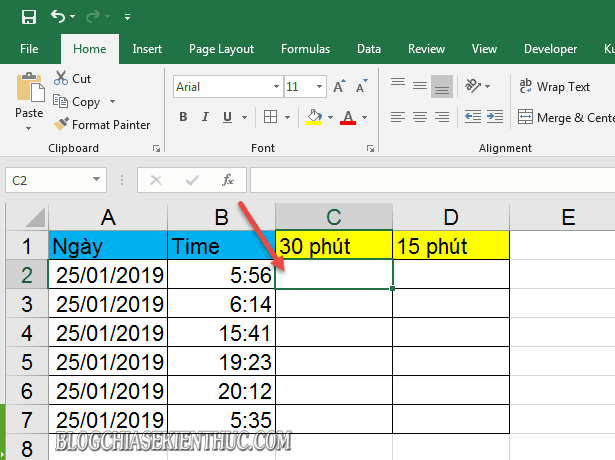+ Step 2: Now enter the formula below => and press `Enter` to execute.

= MOD(INT (B2 / (1/48)) *(1/48);first)

In that box B2 is the time value reference cell to round.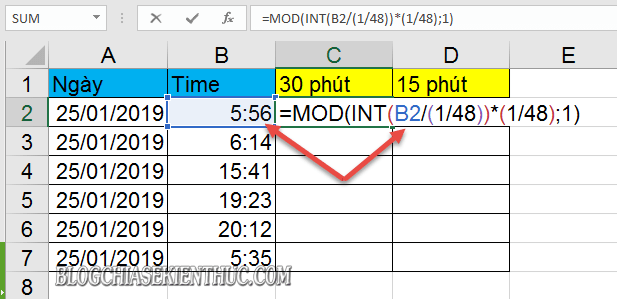+ Step 3: Now we will get valuable results `General` of cell => next you choose the formula cell => and then click on the triangle icon in reverse `Number Format` in the tab `HOME` => and convert the format to `Time` as shown below.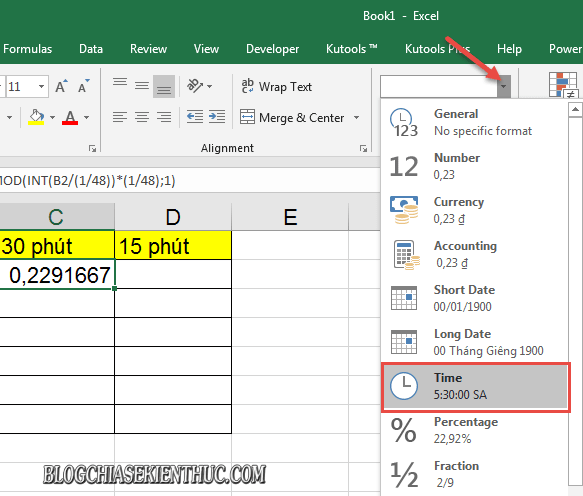Before filling the formula cell down the whole list => and we get the result of rounding the time `30 phút`.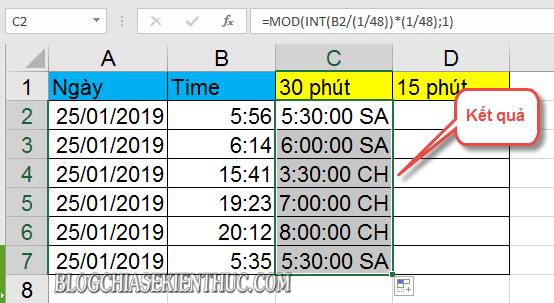### #2. How to use the FLOOR function rounds time to about 15 minutes or set value

In addition to applying the MOD function to round the time value to 30 minutes. For spreadsheet requirements that require rounding to lower values, you can apply the function `FLOOR` in Excel.

Formula formula: = FLOOR(B2;TIME (0, 15, 0))

• Reference value in cell B2, and the 15 minute time value in the range `(1;15;1)`.
• In case you need to round to a value less than 15 minutes, you can edit the value 15 It's about any value of yours. Before pressing Enter to execute.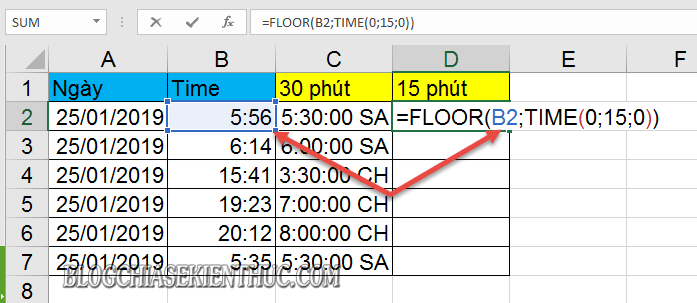Then change the format `General` default to `Time` as shown below.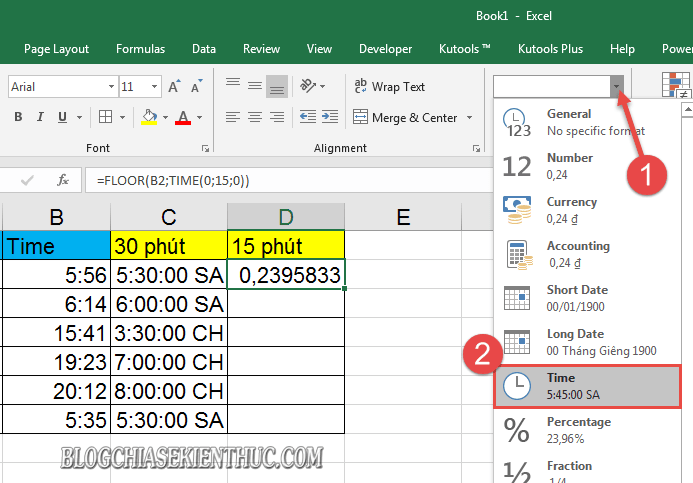Finally, you fill the formula cell down the whole list to get the result of the time value rounded to about 15 minutes.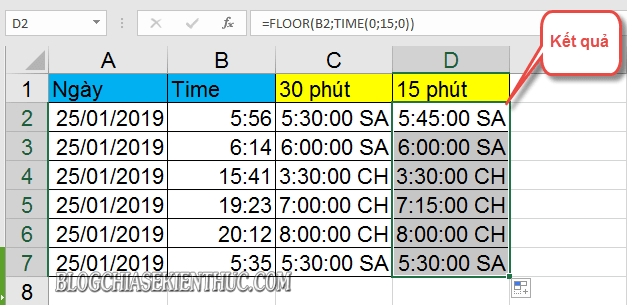### # 3. Epilogue

Okay, that's it! I have just instructed you to add small tricks in using structure MOD and FLOOR functions to round the time value to half an hour, or 15 minutes ... in a very simple way.

Come here then post Instructions for rounding time in Excel I also would like to pause. Hope this tip will be helpful in your work.

Good luck !

CTV: Luong Trung - Blogchiasekienthuc.com